# What is a pn Junction ? Discuss the behaviour of a pn junction under forward and reverse biasing . Explain the V-I Characteristics of a pn Junction

## What Is a PN Junction

When a p-type semiconductor is suitably joined to an n-type semiconductor, the contact surface is called pn junction.

Most semiconductor devices contains one or more pn junctions. The pn junction is of great importance as it is the main control element  for the semiconductor devices.

### Formation of pn Junction

In actual practice, a pn junction will not be formed if a p-type block is just brought in contact with n-type block. In fact, pn junction is fabricated by special techniques.

One common method of making pn junction is known as alloying.

In this method, a small block of indium (trivalent impurity) is placed on an n-type germanium slab as shown in fig.1 (i).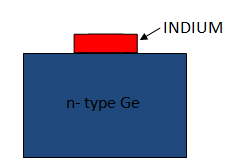Fig.1(i)

The system is then heated to a temperature of about 500o C.

The indium and some of the germanium melt to form a small puddle of molten germanium-indium mixture as shown in fig.1 (ii).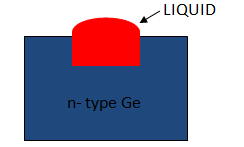Fig.1(ii)

The temperature is then lowered and puddle begins to solidify.

Under proper conditions, the atoms of indium impurity will be suitably adjusted in the germanium slab to form a single crystal.

The addition of indium overcomes the excess of electrons in the n-type germanium to such an extent that it creates a p-type region.

As the process goes on, the remaining molten mixture becomes increasingly rich in indium.

When all the germanium has been redeposited, the remaining material appears as indium button which is frozen on the outer surface of the crystallised portion as shown in fig.1(iii).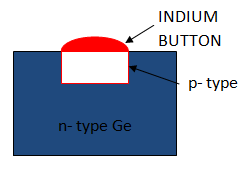Fig.1(iii)

This button serves as a suitable base for soldering on leads.

### Properties of pn Junction

At the instant of pn-junction formation, the free electrons near the junction in the n region begin to diffuse across the the junction into the p region where they combine with holes near the junction.

As a result n region loses free electrons and this creates a layer of positive charges (pentavalent ions) near the junction.

As the electrons move across the junction, the p region loses holes as the electrons and holes combine.

The result is that there is a layer of negative charges ( trivalent ions) near the junction.

These two layer of positive and negative charges form the depletion region or depletion layer.

The term depletion is due to the fact that near the junction, the region is depleted i.e emptied of charge carriers (free electrons and holes) due to diffusion across the junction.

The depletion layer is formed very quickly and is  very thin as compared to the n region and the p region.

Once pn junction is formed and depletion layer is created, the diffusion of free electrons stops.

In other words, the depletion layer acts as a barrier to the further movement of free electrons across the junction.

The positive and negative charges set up an electric field which acts as a barrier to the free electrons in the n region. This is shown in fig.2.Fig.2

There exist a potential difference across the depletion layer known as barrier potential (V0).

The typical barrier potential is approximately:

For silicon, V0= 0.7 V,   For germanium, V0= 0.3 V.

### Biasing a pn Junction

In electronics, the term bias refers to the use of d.c. voltage to establish certain operating conditions for an electronic device.

In case of a pn junction, there are following two bias conditions:

1. Forward biasing
2. Reverse biasing

#### 1. Forward Biasing

When external d.c. voltage applied to the junction is in such a direction that it cancels the potential barrier, thus permitting current flow, it is called forward biasing.

To apply forward bias, the positive terminal of the battery is connected to p-type and negative terminal is connected to n-type of the pn-junction as shown in fig.3.

The applied forward potential establishes an electric field which acts against the field due to potential barrier.

Therefore, the resultant field is weakened and the barrier height is reduced at the junction as shown in fig.3.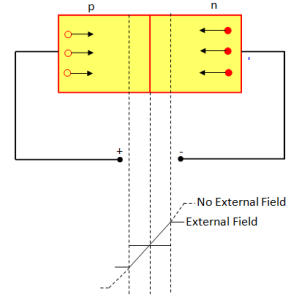Fig.3

As potential barrier voltage is very small (0.1 to 0.3 V ), therefore, a small forward voltage is sufficient to completely eliminate the barrier.

Once the barrier is eliminated by the forward voltage, junction resistance becomes almost zero and a low resistance path is established for the entire circuit.

Therefore, current flows in the circuit. This is called forward current.

#### 2. Reverse Biasing

When the external d.c. voltage applied to the junction is in such a direction that potential barrier is increased, it is called reverse biasing.

To apply reverse bias, the positive terminal of the battery is connected to n-type and negative terminal to p-type of the pn junction as shown in fig.4.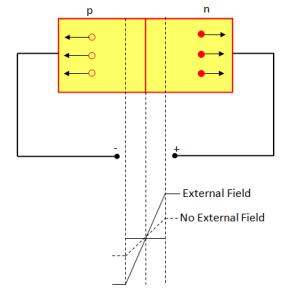Fig.4

The applied reverse voltage establishes an electric field which acts in the same direction as the field due to potential barrier.

Therefore, the resultant field at the junction is strengthened and the barrier height is increased as shown in fig.4.

The increased potential barrier prevents the flow of charge carriers across the junction.

Thus, a high resistance path is established for the entire circuit and hence the current does not flow.

### Current Flow in a Forward Biased pn junction

Fig .5 shows a forward biased pn junction.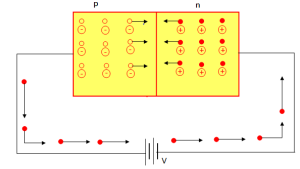Fig.5

Under the influence of forward voltage, the free electrons in n-type move towards the junction, leaving behind positively charged atoms.

However, more electrons arrive from the negative terminal of the battery and enter the n-region to take up their places.

As the free electrons reach the junction, they become valence electron. As valence electron, they move through the holes in the p-region.

The valence electron move towards left in the p-region which is equivalent to holes moving to right.

When the valence electron reach the left end of the crystal, they flow into the positive terminal of the battery.

Summery:

1. The free electrons from the negative terminal continue to pour into the n-region while the free electrons in the n-region move towards the junction.
2. The electrons travel through the n-region as free electrons i.e current in n-region is by free electrons.
3. When these electrons reach the junction, they combine with holes and become valence electrons.
4. The electrons travel through p-region as valence electrons i.e. current in the p-region is by holes.
5. When these valence electrons reach the left end of the crystal, they flow into the positive terminal of the battery.

### V-I Characteristics of pn Junction

Volt-Ampere or V-I characteristics of a pn junction is the curve between voltage across the junction and the circuit current.

Usually voltage is taken across x-axis and current along y-axis.

Fig.6 shows the circuit arrangement for determining the V-I characteristics  of a pn junction.Fig.6

The characteristics can be explained under three conditions namely zero external voltage, forward bias and reverse bias.

#### (i) Zero External Voltage:

When the external voltage is zero, i.e. circuit is open  at K, the potential barrier at the junction does not permit current flow. Therefore, circuit current is zero as indicated by point O in fig.7.Fig.7

#### (ii) Forward Bias:

With forward bias to the pn junction i.e. p-type is connected to positive terminal and n-type is connected to negative terminal, the potential barrier is reduced.

At some forward voltage (0.7 V for Si and 0.3 V for Ge), the potential barrier is altogether eliminated and current starts flowing in the circuit.

From now onwards, the current increases with the increase in forward voltage. Thus a rising curve OB is obtained with forward bias as shown in fig.7.

From the forward characteristics, it is seen that at first (i.e region OA ), the current increase very slowly and curve is non-linear. It is bacause the external applied volateg is used to overcome the potential barrier.

However, once the external applied voltage  exceeds the potential barrier voltage, the pn junction behaves like an ordinary conductor. Therefore, current rises very sharply with increase in voltage (region AB). The curve is almost linear.

#### (iii) Reverse Bias:Fig.8

With reverse bias to the pn junction i.e. p-type connected to negative terminal and n-type connected to positive terminal, potential barrier at the junction is increased.

Therefore, the junction resistance becomes very high and practically no current flows through the circuit.

However, in practice, a very small current (of the order of μA) flows in the circuit with reverse bias as shown in fig.8.

This is called reverse saturation current (Is) and is due to the minority carriers.

To the free electrons in p-type and holes in n-type, the applied reverse bias appears as forward bias. Therefore, a small current flows in the reverse direction.

If the reverse voltage is increased continuously, the kinetic energy of minority carriers may become high enough to knock out electrons from the semiconductor atom. At this stage breakdown of the junction occurs. This is characterised by a sudden rise of reverse current and a sudden fall of the resistance of barrier region. This may destroy the junction permanently.